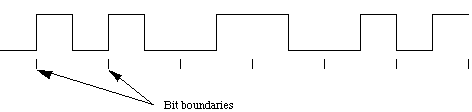# Manchester Encoding

Manchester encoding (first published in 1949) is a synchronous clock-encoding technique used by the physical layer to encode the clock and data of a synchronous bit stream. In this technique, the actual binary data to be transmitted over the cable are not sent as a sequence of logic 1's and 0's (known technically as Non Return to Zero (NRZ)). Instead, the bits are translated into a slightly different format that has a number of advantages over using straight binary encoding (i.e. NRZ).

In the Manchester encoding shown (as defined by IEEE 802.3 standards for 10 Mbps), a logic zero is indicated by a 1 to 0 transition at the centre of the bit and a logic one is indicated by a 0 to 1 transition at the centre of the bit. The signal transitions do not always occur at the ‘bit boundaries’ (the division between one bit and another), but that there is always a transition at the centre of each bit. The Manchester encoding rules are summarised below:

 Original Data Value Sent Logic 0 1 to 0 (downward transition at bit centre) Logic 1 0 to 1 (upward transition at bit centre)

Note that in some cases you will see the encoding reversed, with 0 being represented as a 0 to 1 transition. The two definitions have co-existed for many years. Because many physical layers employ an inverting line driver to convert the binary digits into an electrical signal, the signal on the wire is the exact opposite of that output by the encoder. Differential physical layer transmission, (e.g. 10BT) does not suffer this inversion ambiguity.

The following diagram shows a typical Manchester encoded signal with the corresponding binary representation of the data (0,0,1,0,1,1) being sent.The waveform for a Manchester encoded bit stream carrying the sequence of bits: 001011.

Note that signal transitions do not always occur at the 'bit boundaries' (the division between one bit and another), but that there is always a transition at the centre of each bit.The encoding may be alternatively viewed as a phase encoding where each bit is encoded by a postive 90 degree phase transition, or a negative 90 degree phase transition. The Manchester code is therefore sometimes known as a Biphase Code.

A Manchester encoded signal contains frequent level transitions that allow a receiver to extract the clock signal using a Digital Phase Locked Loop (DPLL) and then correctly decode the value of each bit. To allow reliable operation using a DPLL, the transmitted bit stream must contain a high density of bit transitions. Manchester encoding ensures this, allowing the receiving DPLL to correctly extract the clock signal.

Manchester encoding can consume up to approximately twice the bandwidth of the original signal (20 MHz). This is the penalty for introducing frequent transitions. For a 10 Mbps LAN, the signal spectrum lies between the 5 and 20 MHz. Manchester encoding is used as the physical layer of an Ethernet LAN, where the additional bandwidth is not a significant issue for coaxial cable transmission, the limited bandwidth of CAT5e cable necessitated a more efficient encoding method for 100 Mbps transmission using a 4b/5b MLT-3 code. MLT-3 uses three signal levels (instead of the two levels used in Manchester encoding) and therfore allows a 100 Mbps signal to occupy just 31 MHz of bandwidth. Gigabit Ethernet utilises five levels and 8b/10b encoding, to provide even more efficient use of the limited cable bandwidth, sending 1 Gbps within 100 MHz of bandwidth.

### Example of Manchester Encoding

The pattern of bits " 1 0 0 0 1 0 " encodes to " 01 10 10 10 10 01 01 10".

Here is another example: 1 1 0 0 0 1 after encoding becomes: 010110101001.

### Differential physical layer transmission

Differential physical layer transmission, (e.g., 10BT) transmits both a positive and negative version of the signals. This does not suffer this inversion in Manchester encoding, since the polarity of the data bits can be determined from the polarity of the Start of Frame Delimiter (SFD).

### Bi-Phase Encoding

Manchester encoding may be alternatively viewed as a phase encoding where each bit is encoded by a positive 90 degree phase transition, or a negative 90 degree phase transition. The Manchester code is therefore sometimes known as a Biphase Code.

### Thinking more about sending bits

A transmission rate of 10 Mbps requires that each bit is sent in 0.1 microseconds. For a coaxial cable, the speed at which the signal travels along the cable is approximately 0.77 times the speed of light (i.e. 0.77x3x10E8). A bit therefore occupies 23 metres of cable. Under the same conditions the smallest frame would be 13.3 km!

If you wish to do the same calculation for a twisted pair cable, you would have to take into consideration that the propagation speed is slower at 1.77x10E8 (0.59c). Increasing the bit rate, for example using 100BTx, decreases the time available to send each bit into the wire, but does not change the speed at which the edge of the bits travel through the cable!

Gorry Fairhurst - Date: 26/02/2020 EE3567